# CASE statement in SQL Server: T-SQL Example

## Overview of Case in real life!

Again, in real life, we perform different actions depending upon the outcome of different conditions.

To elaborate more, consider below example:

• If flight tickets are less than \$100, then I will visit Los Angeles.
• If flight tickets are between \$100 to \$200, then I will visit New York
• If flight tickets are between \$200 to \$400, then I will visit Europe
• Else, I will prefer to visit some nearby tourist spot.

Let’s consider categorizing Condition and Action separately from the above example below:

 Conditions – Flight Tickets Actions performed, only if Condition is TRUE Less than \$100 Visit Los Angeles Between \$100 to \$200 Visit New York Between \$200 to \$400 Visit Europe None of the above condition met Nearby tourist spot

In the above example, we can see that the outcome of the different conditions is governing separate action. E.g., Visitor will perform the act of visiting New York only in the condition if the flight ticket is between \$100 to \$200.

Similarly, MS SQL CASE statement also provides the capability to take action of executing different T-SQL statement based upon the outcome of different conditions.

In this tutorial, you will learn:

## What is CASE?

CASE is the extension of IF…ELSE statement. Unlike IF…ELSE, where only the maximum of one condition is allowed, CASE allows the user to apply multiple conditions to perform different sets of actions in MS SQL. Let’s learn this concept in detail in the following sections.

In MS SQL, there are two types of CASE.

1. Simple CASE
2. Searched CASE

## Simple CASE

#### The syntax for Simple Case

```CASE <Case_Expression>
WHEN Value_1 THEN Statement_1
WHEN Value_2 THEN Statement_2
.
.
WHEN Value_N THEN Statement_N
[ELSE Statement_Else]
END AS [ALIAS_NAME]
```

Here,

• The parameter Case_Expression denotes the expression which we will eventually be compared to Value_1, Value_2, …
• The parameters Statement_1, Statement_2… denote the Statements which will be executed if Case_Expression = Value_1, Case_Expression = Value_2, … and so on.
• In a nutshell, the condition is whether Case_Expression = Value_N and ACTION is the execution of Statement_N if the above result is TRUE.
• ALIAS_NAME is optional and is the alias name given to CASE statement result. Mostly used when we use CASE in the select clause.

### Rules for Simple Case:

• Simple Case only allows equality check of Case_Expression with Value_1 to Value_N.
• The Case_Expression is compared with Value, in order starting from the first value, i.e., Value_1. Below is the execution approach:
• If Case_Expression is equivalent to Value_1, then further WHEN…THEN statements are skipped, and CASE execution will END immediately.
• If Case_Expression does not match with Value_1, then Case_Expression is compared with Value_2 for equivalency. This process of comparing Case_Expression with Value will continue until Case_Expression finds matching equivalent value from the set of Value_1, Value_2,…
• If nothing matched, then control goes to ELSE statement, and Statement_Else will get executed.
• ELSE is optional.
• If ELSE is not present and Case_Expression matches with none of the values, then Null will be displayed.

Below Diagram illustrate the execution flow of Simple Case.

### Examples:

Assumption: Assume that we have the table as ‘Guru99’ with two columns and four rows as displayed below:We will use ‘Guru99’ table in further examples

Query 1: SIMPLE CASE with the NO ELSE option

```SELECT Tutorial_ID, Tutorial_name,
CASE Tutorial_name
WHEN 'SQL' THEN 'SQL is developed by IBM'
WHEN 'PL/SQL' THEN 'PL/SQL is developed by Oracle Corporation.'
WHEN 'MS-SQL' THEN 'MS-SQL is developed by Microsoft Corporation.'
END AS Description
FROM Guru99
```

Result: Below diagram explains the execution flow of a SIMPLE CASE with NO ELSE.

Query 2: SIMPLE CASE with the ELSE option.

```SELECT Tutorial_ID, Tutorial_name,
CASE Tutorial_name
WHEN 'SQL' THEN 'SQL is developed by IBM'
WHEN 'PL/SQL' THEN 'PL/SQL is developed by Oracle Corporation.'
WHEN 'MS-SQL' THEN 'MS-SQL is developed by Microsoft Corporation.'
ELSE 'This is NO SQL language.'
END AS Description
FROM Guru99
```

Result: Below diagram explains the execution flow of a SIMPLE CASE with ELSE.## Searched CASE

The syntax for Searched Case

```CASE
WHEN <Boolean_Expression_1> THEN Statement_1
WHEN <Boolean_Expression_2> THEN Statement_2
.
.
WHEN <Boolean_Expression_N> THEN Statement_N
[ELSE Statement_Else]
END AS [ALIAS_NAME]
```

Here,

• The parameter Boolean_Expression_1, …denotes the expression which will be evaluated for TRUE or FALSE.
• The parameters Statement_1, Statement_2… denote the Statements which will execute if its corresponding Boolean_Expression_1, Boolean_Expression_2 result is TRUE.
• In a nutshell, Condition is Boolean_Expression_1,… and ACTION is the execution of Statement_N if above boolean_Expression_1 is TRUE.
• ALIAS_NAME is optional and is the alias name given to CASE statement result. Mostly used when we use CASE in the select clause.

### Rules for Searched Case:

• Unlike the simple case, Searched Case is not restricted to only equality check but allows Boolean expression.
• The Boolean expression is evaluated, in order starting from the first Boolean expression, i.e., Boolean_expression_1. Below is the execution approach:
• If Boolean_expression_1 is TRUE, then further WHEN…THEN statements are skipped, and CASE execution will END immediately.
• If Boolean_expression_1 is FALSE, then Boolean_expression_2 is evaluated for TRUE condition. This process of assessing Boolean_expression will continue until one of the Boolean_expression returns TRUE.
• If nothing matched, then control goes to ELSE statement, and Statement_Else will get executed.
• Like Simple Case ELSE is optional in Search case as well.
• If ELSE is not present and none of the Boolean_expression return TRUE, then Null will be displayed.

Below Diagram illustrate the execution flow of the Searched Case.

### Examples:

Query 1: SEARCHED CASE with the NO ELSE option

```SELECT Tutorial_ID, Tutorial_name,
CASE
WHEN Tutorial_name = 'SQL' THEN 'SQL is developed by IBM'
WHEN Tutorial_name = 'PL/SQL' THEN 'PL/SQL is developed by Oracle Corporation.'
WHEN Tutorial_name = 'MS-SQL' THEN 'MS-SQL is developed by Microsoft Corporation.'
END AS Description
FROM Guru99
```

Result: Below diagram explains the execution flow of the SEARCHED CASE with NO ELSE.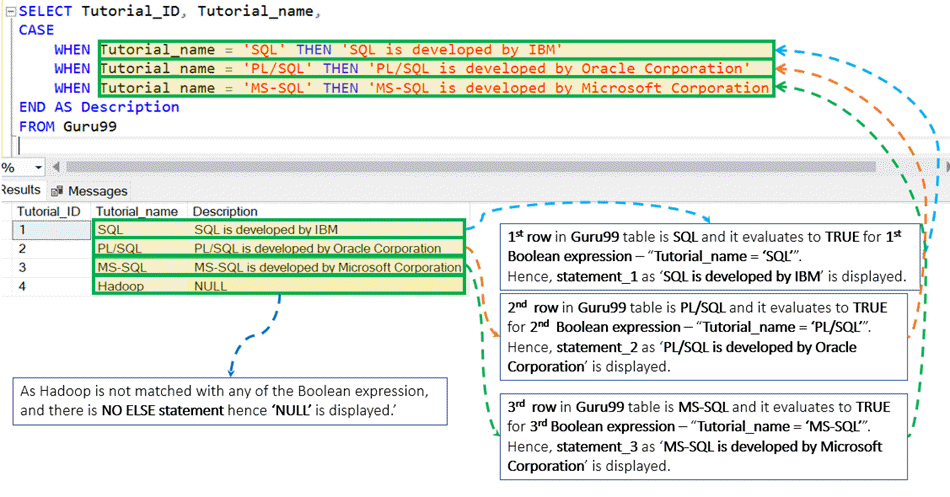Query 2: SEARCHED CASE with the ELSE option.

```SELECT Tutorial_ID, Tutorial_name,
CASE
WHEN Tutorial_name = 'SQL' THEN 'SQL is developed by IBM'
WHEN Tutorial_name = 'PL/SQL' THEN 'PL/SQL is developed by Oracle Corporation.'
WHEN Tutorial_name = 'MS-SQL' THEN 'MS-SQL is developed by Microsoft Corporation.'
ELSE 'This is NO SQL language.'
END AS Description
FROM Guru99
```

Result: Below diagram explains the execution flow of the SEARCHED CASE with ELSE.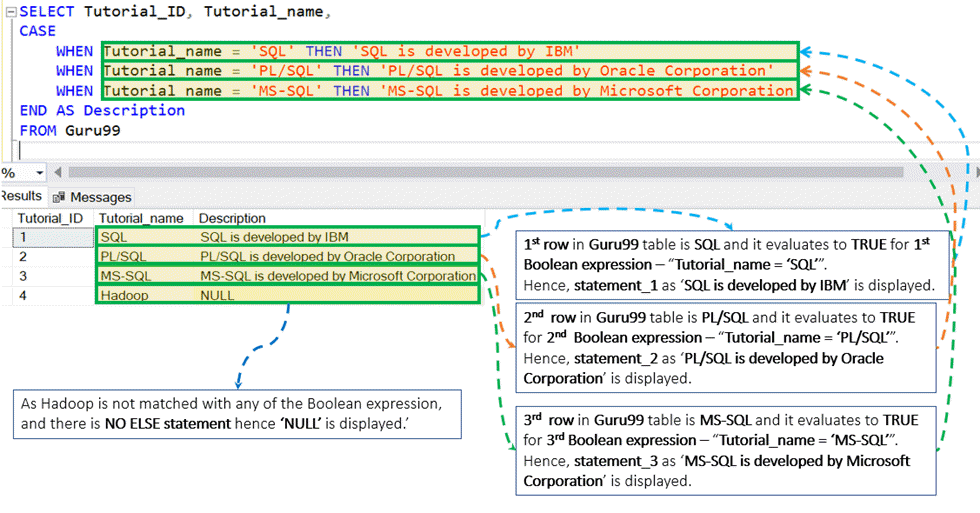### Difference between execution approach: SIMPLE and SEARCH CASE.

Let’s have a look at SIMPLE CASE example below:

```SELECT Tutorial_ID, Tutorial_name,
CASE Tutorial_name
WHEN 'SQL' THEN 'SQL is developed by IBM'
WHEN 'PL/SQL' THEN 'PL/SQL is developed by Oracle Corporation.'
WHEN 'MS-SQL' THEN 'MS-SQL is developed by Microsoft Corporation.'
ELSE 'This is NO SQL language.'
END AS Description
FROM Guru99
```

Here, ‘Tutorial_name’ is a part of CASE expression. Then ‘Tutorial_name’ value is compared with each WHEN values, i.e. ‘SQL’… until ‘Tutorial_name’ matches with WHEN values.

On Contrary, SEARCH CASE example has no CASE Expression:

```SELECT Tutorial_ID, Tutorial_name,
CASE
WHEN Tutorial_name = 'SQL' THEN 'SQL is developed by IBM'
WHEN Tutorial_name = 'PL/SQL' THEN 'PL/SQL is developed by Oracle Corporation.'
WHEN Tutorial_name = 'MS-SQL' THEN 'MS-SQL is developed by Microsoft Corporation.'
END AS Description
FROM Guru99
```

Here, each WHEN statement has its Conditional Boolean expression. Each Boolean expression i.e. Tutorial_name = ‘SQL’,… is evaluated for TRUE/FALSE until first Boolean expression which evaluates to TRUE.

## Difference between Simple and searched case

 Simple Case Searched Case CASE keyword is immediately followed by CASE_Expression and before WHEN statement.E.g.:CASE WHEN Value_1 THEN Statement_1… Case keyword is followed by the WHEN statement, and there is no expression between CASE and WHEN.E.g.:CASE WHEN THEN Statement_1… In Simple Case, VALUE exists for each WHEN statement. This Values: Value_1, Value_2… Are compared with single CASE_Expression sequentially. The result gets evaluate for the TRUE/FALSE condition for each WHEN Statement.E.g.:CASE WHEN Value_1 THEN Statement_1…WHEN Value_2 THEN Statement_2… In Searched Case, Boolean_Expression exists for each WHEN statement. This Boolean_Expressions: Boolean_Expression_1, Boolean_Expression_2,… evaluates the TRUE/FALSE condition for each WHEN Statement.E.g.:CASEWHEN THEN Statement_1…WHEN THEN Statement_2… Simple Case support only equality check. I.e. whether CASE_Expression = VALUE_1, VALUE_2…E.g.:CASE WHEN Value_1 THEN Statement_1…In the above example, the only operation performed by the system is checking if Case_Expression = Value_1 With Boolean_Expression_N, Search Case support any operation which results in a Boolean value. It includes equal and not equal to operator.E.g.:CASE WHEN THEN Statement_1… In above example, Boolean_Expression_1 can contain both ‘equal to’ and ‘not equal to’ operator like A = B, A != B.

## Nested CASE: CASE in IF ELSE

We can use CASE inside IF ELSE. Below is the example MS-SQL code

```DECLARE @Flight_Ticket int;
SET @Flight_Ticket = 190;
IF @Flight_Ticket > 400
PRINT 'Visit Nearby Tourist Location';
ELSE
BEGIN
SELECT
CASE
WHEN @Flight_Ticket BETWEEN 0 AND 100 THEN 'Visit Los Angeles'
WHEN @Flight_Ticket BETWEEN 101 AND 200 THEN 'Visit New York'
WHEN @Flight_Ticket BETWEEN 201 AND 400 THEN 'Visit Europe'
END AS Location
END
```

In the above example CASE is NESTED inside IF…ELSE statement:

First, IF Statement will execute and in case Condition is False then ELSE statement will execute.

Else contain Nested CASE inside it. Depending upon Flight ticket value, one amongst the following result will be displayed:

• The system will print ‘Visit Nearby Tourist Location’ if flight tickets are > \$400
• The system will print ‘Visit Los Angeles’ if flight tickets are BETWEEN \$0 AND \$100
• The system will print ‘Visit New York’ if flight tickets are BETWEEN \$101 AND \$200
• The system will print ‘Visit Europe’ if flight tickets are BETWEEN \$201 AND \$400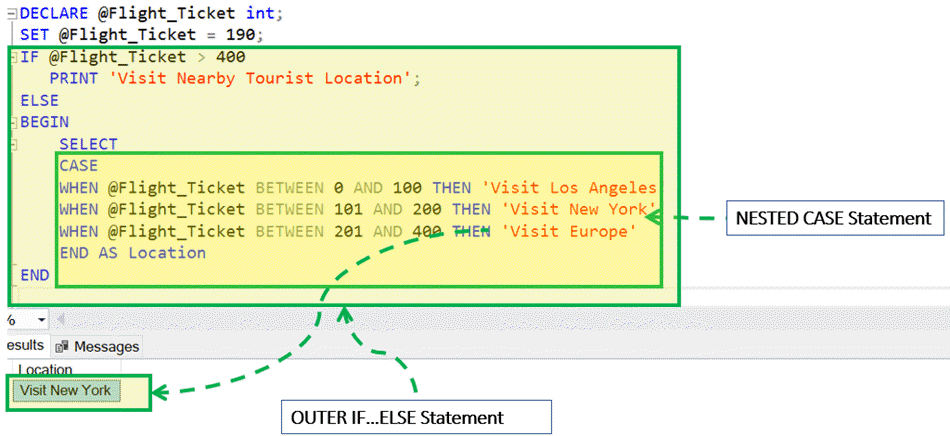## Nested CASE: CASE inside CASE

We can use CASE inside CASE. Below is the example MS-SQL code

```DECLARE @Flight_Ticket int;
SET @Flight_Ticket = 250;
SELECT
CASE
WHEN @Flight_Ticket >= 400 THEN 'Visit Nearby Tourist Location.'
WHEN @Flight_Ticket < 400 THEN
CASE
WHEN @Flight_Ticket BETWEEN 0 AND 100 THEN 'Visit Los Angeles'
WHEN @Flight_Ticket BETWEEN 101 AND 200 THEN 'Visit New York'
WHEN @Flight_Ticket BETWEEN 201 AND 400 THEN 'Visit Europe'
END
END AS Location
```

In the above example CASE is NESTED inside another CASE statement:

The system starts with executing the outer CASE. If Flight_Ticket < \$400 then inner CASE will execute.

Depending upon Flight ticket value, one amongst the following result will be displayed:

• The system will print ‘Visit Nearby Tourist Location’ if flight tickets are > \$400
• The system will print ‘Visit Los Angeles’ if flight tickets are BETWEEN \$0 AND \$100
• The system will print ‘Visit New York’ if flight tickets are BETWEEN \$101 AND \$200
• The system will print ‘Visit Europe’ if flight tickets are BETWEEN \$201 AND \$400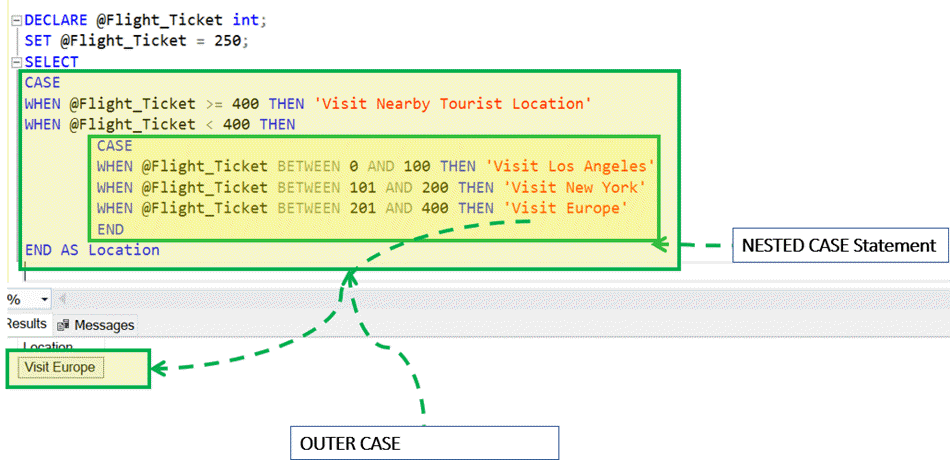## CASE with UPDATE

Assumption: Assume that we have the table as ‘Guru99’ with two columns and four rows as displayed below: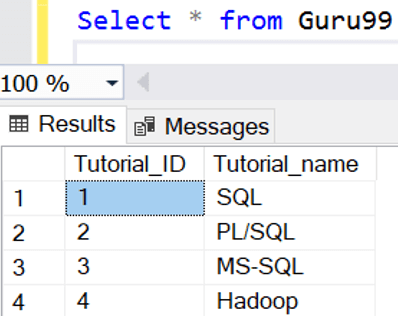We will use ‘Guru99’ table in further examples

We can use CASE with UPDATE. Below is the example MS-SQL code:

```UPDATE Guru99
SET Tutorial_Name =
(
CASE
WHEN Tutorial_Name = 'SQL' THEN 'Structured Query language.'
WHEN Tutorial_Name = 'PL/SQL' THEN 'Oracle PL/SQL'
WHEN Tutorial_Name = 'MSSQL' THEN 'Microsoft SQL.'
END
)
```

In the above example CASE is used in the UPDATE statement.

Depending upon Tutorial_Name Value, Tutorial_Name column will get the update with THEN Statement value.

• If Tutorial_Name = ‘SQL’ THEN update Tutorial_Name to ‘Structured Query language’
• If Tutorial_Name = ‘PL/SQL’ THEN update Tutorial_Name to ‘Oracle PL/SQL’
• If Tutorial_Name = ‘MSSQL’ THEN update Tutorial_Name to ‘Microsoft SQL’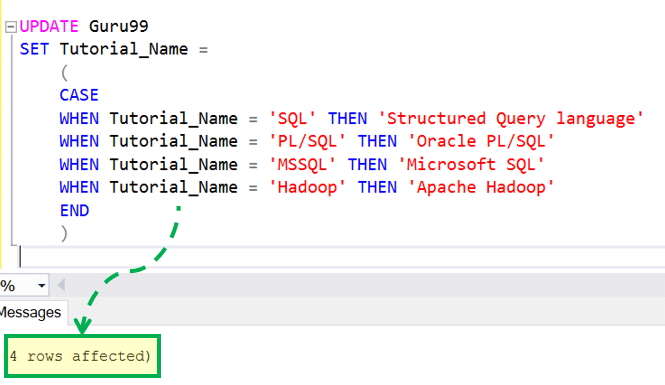Let’s Query Guru99 table to check the updated value: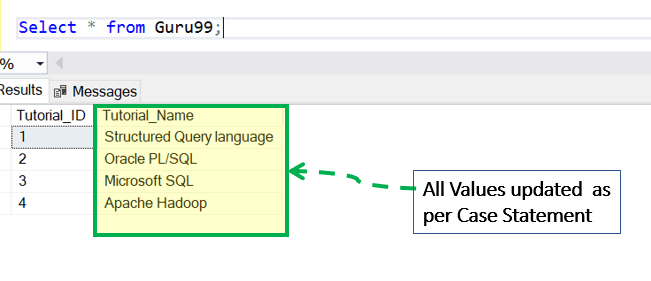## CASE with Order by

We can use CASE with Order By. Below is the example MS-SQL code:

```Declare @Order Int;
Set @Order = 1
Select * from Guru99 order by
CASE
WHEN @Order = 1 THEN Tutorial_ID
WHEN @Order = 2 THEN Tutorial_Name
END
DESC
```

Here CASE is used with Order By.

@Order is set to 1 and as first WHEN Boolean expression evaluates to TRUE, Tutorial_ID is selected for Order by Condition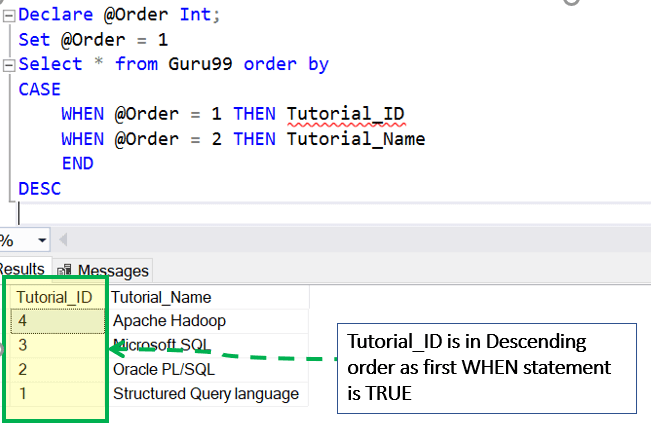## Interesting Facts!

• CASE can be nested in another CASE as well as in another IF…ELSE statement.
• In addition to SELECT, CASE can be used with another SQL clause like UPDATE, ORDER BY.

## Summary:

• In MS SQL, there are two types of CASE: Simple CASE and Searched CASE
• ELSE is optional in the CASE statement.

#### Top Courses